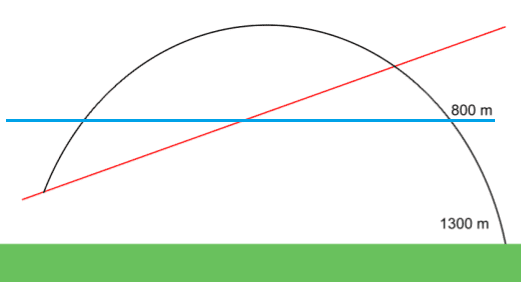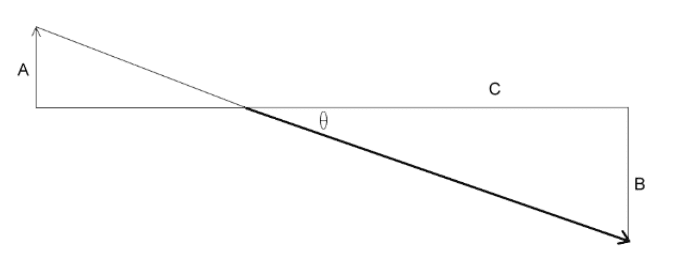# Bullet drop relative to line of sight

I'm kind of confused by this:

Suppose a rifle is zeroed in at 800 meters (the bullet hits the line of sight at 800m).

This means the bullet must be fired at an upwards angle, the scope stays pointing directly at 800 meters.

Crude diagram, line of sight = blue, barrel direction = red, not to scale at allI want to find the drop relative to the line of sight.

Say the total bullet drop (at 1300 meters) is 33 meters.

The total drop at the 800 meter mark is 8 meters (bullet exactly on line of sight)

I figured the total drop relative to the line of sight would be 33 minus 8 = 25 meters remaining.

However it is only 14 meters, according to my ballistic calculator... another example has total drop = 40 meters, drop at 800 = 8 meters, drop relative to line of sight = 20 meters (not the 32 I would have supposed).

So I'm obviously missing something simple here...

mfb
Mentor
Your red and blue line start nearly at the same position, right? I would expect just ~10cm between them, this difference should be negligible.

Say the total bullet drop (at 1300 meters) is 33 meters.

The total drop at the 800 meter mark is 8 meters (bullet exactly on line of sight)
Where do those numbers come from? They don't look consistent.

Anyway, the red and blue line increase their distance between 800 and 1300m. They deviate by 8 m at 800m (right?), so they should be roughly 8m*1300/800=13m apart at 1300m.

Andrew Mason
Homework Helper
I want to find the drop relative to the line of sight.

Say the total bullet drop (at 1300 meters) is 33 meters.

The total drop at the 800 meter mark is 8 meters (bullet exactly on line of sight)

I figured the total drop relative to the line of sight would be 33 minus 8 = 25 meters remaining.

However it is only 14 meters, according to my ballistic calculator... another example has total drop = 40 meters, drop at 800 = 8 meters, drop relative to line of sight = 20 meters (not the 32 I would have supposed).
No. That 8 m at 800 m. range becomes 13 m. at 1300 m. range. But you also have to factor in a loss of speed between 800 m and 1300 m. and the fact that the bullet is accelerating so its average downward speed is much greater between 800 and 1300 m than it was between 0-800 m.

Here is how it works:

The drop is: Δs = gt2/2 where g = 9.8 m.sec-2. This is very accurate if the drop << range.

The time of flight is determined by the speed of the bullet. Over 800 m the bullet is going to slow down significantly. You have to experiment.

If the drop is 8 m over a range of 800 m, then the time of flight is: $t = \sqrt{2Δs/g} = \sqrt{16/9.8} = 1.3 s$

This means that the average speed is v = d/t = 800/1.3 = 615 m/sec or about 2000 fps.

So you would zero the scope so that the scope is pointing at an angle of arctan 8/800 = .57 degrees below the direction of the barrel. With this angle, by putting the target in the cross-hairs of the scope you will hit the target at 800 m. range (assuming the 8 m drop over 800 m. is accurate).

Now, if you are aiming at a target 1300 m. away and you have determined from experiment that the drop is 33 m., this means that the time of flight over 1300 m is: $t = \sqrt{2Δs/g} = \sqrt{66/9.8} = 2.6 s$. So the average speed over 1300 m is v = d/t = 1300/2.6 = 500 m/sec.

To hit this target you would have to aim the barrel at an angle of arctan 33/1300 = 1.45 degrees above the target. But if you have the scope already aiming .57 degrees below the direction of the barrel, you will have to aim the scope only 1.45-.57=.88 degrees above the target. This means you would aim through the scope at a point that is 1300tan(.88) = 19.9 metres above the target.

AM

Last edited:
Baluncore
2021 Award
Without drag from air resistance the bullet would travel along an inverted parabola. But drag is dependent on bullet profile and airspeed. The drag function is normally given as a table of data for a particular design.

The subject here is called Exterior or External Ballistics.
http://en.wikipedia.org/wiki/External_ballistics

Andrew Mason
Homework Helper
Without drag from air resistance the bullet would travel along an inverted parabola. But drag is dependent on bullet profile and airspeed. The drag function is normally given as a table of data for a particular design.

The subject here is called Exterior or External Ballistics.
http://en.wikipedia.org/wiki/External_ballistics
True, but the parabola is quite flat. I was assuming that the drop measurements were made from the horizontal: i.e. the gun pointing horizontally. The analysis is valid only for essentially horizontal shots ie drop << range.

AM

Hmmm, the above works for closer shots with a flat trajectory but I am still confused about longer ranges and more curved trajectories...

Here is an example, with answer, from a ballistics program.

Zero range = 300m

Drop at 300m = 0.655m
Drop at 1922m = 37.491m

Path relative to line of sight at 300m = ZERO
Path relative to line of sight at 1376m = -24.35 meters

So...

1376*tan(arctan(37.49/1376) - arctan(0.655/300)) = 34.48m

Which is an error of over 40%. I put the height of the sights, etc, at zero to make it simpler.

So what do I do when the trajectory is curved? I would like to get the 24.35 answer.

====

Edit: from playing around with it, I see the initial angle of firing has a very large effect on the results... so how do I calculate and allow for the angle of firing?

Ideally I would like to calculate the required angle to hit a target at "X" meters. I suppose I will need to create an Excel spreadsheet or something...

Last edited:
Andrew Mason
Homework Helper
Hmmm, the above works for closer shots with a flat trajectory but I am still confused about longer ranges and more curved trajectories...

Here is an example, with answer, from a ballistics program.

Zero range = 300m

Drop at 300m = 0.655m
Drop at 1922m = 37.491m

Path relative to line of sight at 300m = ZERO
Path relative to line of sight at 1376m = -24.35 meters

So...

1376*tan(arctan(37.49/1376) - arctan(0.655/300)) = 34.48m

Which is an error of over 40%. I put the height of the sights, etc, at zero to make it simpler.

So what do I do when the trajectory is curved? I would like to get the 24.35 answer.

====

Edit: from playing around with it, I see the initial angle of firing has a very large effect on the results... so how do I calculate and allow for the angle of firing?

Ideally I would like to calculate the required angle to hit a target at "X" meters. I suppose I will need to create an Excel spreadsheet or something...

What does the drop at 1922 m have to do with this? Did you mean 1376?

Using your figures, the angle of the scope to the barrel will be:

θs-b = arctan(.655/300) = .125 deg.

The calculator predicts that the angle of the target to the scope sight at 1376 m. will be :

θt-s = arctan(24.35/1376) = 1.014 deg.

So the calculated angle of the target to barrel is:

θt-b = 1.014 + .125 = 1.139 deg.

So the actual calculated drop is 1376tan(1.139) = 27.4 m.

If the actual drop at 1376 m. was 37.491 m this simply means that your bullet is travelling more slowly than the calculator is predicting. The calculator makes some assumptions about bullet aerodynamics and stability.

You need to know the actual drop at each distance. This is a function of the time of flight. If you know how long it takes for the bullet to travel those 1376 metres you can work out the angle. I expect, however, that this is not consistent. It depends on factors that may differ from shot to shot (due to bullet instability, which affects speed). So for these very long distances, the drop at a given range may vary significantly from shot to shot.

AM

Baluncore
2021 Award
The difference between real-world ballistics and the simple-physics model is the drag due to air resistance. Deceleration due to atmospheric drag on a bullet is typically 100 times greater than the falling acceleration due to gravity. The integration of that difference leads to diverging predictions.

If you want the physics equations to match the real world ballistics, you will need to include a “velocity : drag” table for the actual projectile being modelled. You will then have a “ballistics program”.

I am considering the drag, however the vertical drop was off.

I wasn't factoring in that after it is fired horizontally it will have a downwards angle and therefore the acceleration due to air resistance will also affect the bullet in the y-direction, acting against acceleration due to gravity, reducing the drop...For example in the exterior ballistics program a certain bullet is predicted to drop 4.33 meters in 1.09 seconds, while I would calculate 1.09^2*9.80665/2 = 5.80 meters, getting a wrong answer.

Andrew Mason
Homework Helper
I am considering the drag, however the vertical drop was off.

I wasn't factoring in that after it is fired horizontally it will have a downwards angle and therefore the acceleration due to air resistance will also affect the bullet in the y-direction, acting against acceleration due to gravity, reducing the drop...For example in the exterior ballistics program a certain bullet is predicted to drop 4.33 meters in 1.09 seconds, while I would calculate 1.09^2*9.80665/2 = 5.80 meters, getting a wrong answer.
The bullet is not being fired at a downward angle. It is being fired horizontally or at a slight upward angle. You are not accounting for the initial upward speed due to that angle.

The component of air resistance in the vertical direction is not going to have a material effect. Its vertical speed will be vy = v0y - gt where v0y is the initial vertical component of its speed (0 or slightly positive). So after two seconds the vertical speed will be a maximum 64 feet/sec, hardly enough to generate much air resistance.

The calculation for vertical position will be y = y0 + v0sinθt - gt2/2 where v0 is the launch speed (θ being the launch angle - the angle of the gun above the horizontal) and y0 is the height of the muzzle above the ground. It is the v0sinθt term that you have not taken into account.

AM